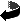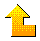Statistics 578: Regression Analysis

 Professor Douglas Wiens Office: CAB 429 Email: doug.wiens@ualberta.ca Reload to change picture

## Course materials:

• Course information, assessment procedures, etc. See also the legal stuff to be included with outlines.
• Schedule of lectures, assignments and exams.
• Lecture notes. These are constantly under revision.
• The course assumes an undergraduate knowledge of Regression at the level of STAT 378, and mathematics at the level of STAT 512. If you are not currently taking 512, you should at least work through the 512 lecture notes – especially the parts on linear algebra and matrix theory (including matrix differentiation), and asymptotic distribution theory. A summary of the required material is in this appendix.

## Obtaining and using the R package:

• For a quick start you can obtain R here and install it on a PC by double-clicking on this .exe file and following the instructions. There is a Mac version of R too; you'll need to consult the 'R home page' link below for this.
• R manuals: small, big.

## Technical Writing:

• The importance of clarity of exposition, and of grammatical correctness, in technical writing cannot be over-emphasized. The best way to determine if you understand what you are doing is to try to write it down in a form that another reader can understand. Some comments along these lines are included with the course information; for some helpful resources see "Writing Aids" on my homepage.

R examples worked in class: You should find it useful to download and run these R scripts before the classes in which they are to be discussed.

• cars (Lecture 1) Some preliminary regression fits
• acetylene1 (Lecture 4) and acetylene 2 (Lecture 5) An analysis of a dataset exhibiting extreme multicollinearity; ridge regression
• logistic (Lecture 6) Example of logistic regression through wls
• outlier (Lecture 7) Simulated example of the effect of one highly influential outlier
• puromycin (Lecture 8) Fitting a Michaelis-Menten model to the puromycin data
• bod1 (Lecture 9) Likelihood regions and profile plots for the BOD data
• lubricant (Lecture 10) Some methods of obtaining starting values
• smoothing1 (Lecture 12) Smoothing spline (and other) fits to the motorcycle data
• smoothing2 (Lecture 13) Kernel and loess fitting
• rock (Lecture 14) GAM and projection pusuit fitting on the 'rock' data set
• ppreg (Lecture 14) Simulated projection pursuit example
• lasso (Lecture 15) Computing the lasso
• quantile regression (Lecture 15); see the Koenker paper
• ordinary M-estimation (Lectures 17, 18) Computing an Ordinary M-estimate with Huber's psi function
• mineral (Lectures 20 -- 23) Various regression fits to the 'mineral' dataset from Maronna, Martin & Yohai
• my GM estimator (Lecture 22) My function to compute GM-estimates

Matlab code

## Data sets used in assignments:

• pcb (Assignment 1)
• bod2 (Assignment 2)
• steady state (Assignment 2)
• water (Assignment 3): download water.dat and water.R to the same directory, and run water.R.  Check that all 20 cases have appeared.

## Statistics resources at U of A:Homepage of Doug WiensDepartment of Mathematical and Statistical Sciences Home Page